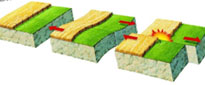#### Warning!

Javascript is disabled on this browser.
Javascript must be enabled for this website to display and function correctly.

# What is earthquake magnitude?

It is a measure of earthquake size and is determined from the logarithm of the maximum displacement or amplitude of the earthquake signal as seen on the seismogram, with a correction for the distance between the focus and the seismometer. This is necessary as the closer the seismometer is to the earthquake, the larger the amplitude on the seismogram, irrespective of the size or magnitude of the event. Since the measurement can be made from P, S or surface waves, several different scales exist, all of which are logarithmic because of the large range of earthquake energies (for example a magnitude 6 ML is 30 times larger, in terms of energy than a magnitude 5 ML). The Richter local magnitude (ML) is defined to be used for 'local' earthquakes up to 600 km away, and is the magnitude scale used by BGS when locating UK earthquakes.

Surface wave magnitude (Ms) is based on the maximum amplitude of the surface wave having a period of 20 + 2 s. It is used for observations near the earthquake epicentre where the surface wave is larger than the body wave. This scale applies to any epicentral distance or type of seismograph.

Body wave magnitude (mb) is calculated from the body waves (P,PP,S) and are usually used at larger distance from the earthquake epicentre (P-wave attenuation is less than surface waves, with distance). It can be used for any earthquake of any depth.

Moment magnitude (Mw) is considered the best scale to use for larger earthquakes as the Ms saturates at about magnitude 8. Moment magnitude is measured over the broad range of frequencies present in the earthquake wave spectrum rather than the single frequency sample that the other magnitude scales use.

For comparison purposes, a magnitude 5 ML earthquake is equivalent to the explosion of 1,000 tons of TNT whereas a magnitude 6 ML earthquake is the energy equivalent of 30,000 tons of TNT or a 30 kilotonne nuclear explosion.